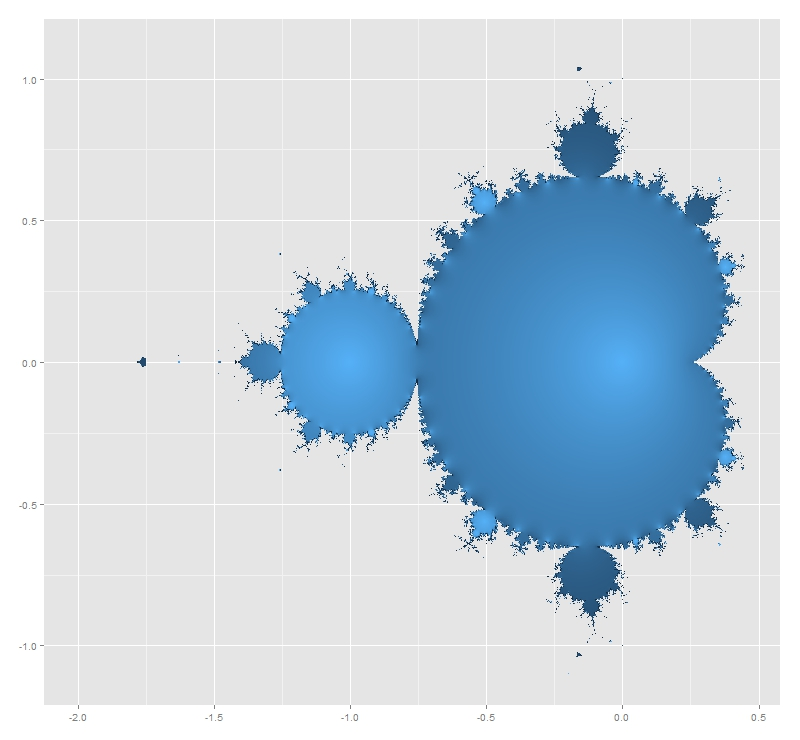# I Need A New Computer To Draw Fractals!

Computer Science is no more about computers than astronomy is about telescopes (E. W. Dijkstra)

Some days ago I published a post about how to build fractals with R using Multiple Reduction Copt Machine (MRCM) algorithm. Is that case I used a feature of the grid package that allows you to locate objects easily into the viewPort avoiding to work with coordinates. It does not work well if you want to divide your seed image into five subimages located in the vertex of a regular pentagon. No problem: after refreshing some trigonometric formulas and after understanding how to work with coordinates I felt strong enough to program the Final-MRCM-Fractal-Builder. But here comes the harsh reality. My computer crashes when I try to go beyond five degrees of depth. Imposible. In the example of Sierpinski’s triangle, where every square in divided into three small ones, I reached seven degrees of depth. I am deeply frustrated. These are drawings for 1, 2, 3 and 5 degrees of depth.

Please, if someone modifies code to make it more efficient, let me know. I used circles in this case instead squares. Here you have it:

```library(grid)
grid.newpage()
rm(list = ls())
ratio <- 0.4
pmax <- 5 # Depth
vp1 <- viewport(w=1, h=1)
vp2 <- viewport(w=ratio, h=ratio, just=c(0.75*sin(2*pi*1/5)+0.5, 0.75*cos(2*pi*1/5)+0.75*pi*1/5))
vp3 <- viewport(w=ratio, h=ratio, just=c(0.75*sin(2*pi*0/5)+0.5, 0.75*cos(2*pi*0/5)+0.75*pi*1/5))
vp4 <- viewport(w=ratio, h=ratio, just=c(0.75*sin(2*pi*2/5)+0.5, 0.75*cos(2*pi*2/5)+0.75*pi*1/5))
vp5 <- viewport(w=ratio, h=ratio, just=c(0.75*sin(2*pi*3/5)+0.5, 0.75*cos(2*pi*3/5)+0.75*pi*1/5))
vp6 <- viewport(w=ratio, h=ratio, just=c(0.75*sin(2*pi*4/5)+0.5, 0.75*cos(2*pi*4/5)+0.75*pi*1/5))
pushViewport(vp1)
grid.rect(gp=gpar(fill="white", col=NA))
m <- as.matrix(expand.grid(rep(list(2:6), pmax)))
for (j in 1:nrow(m))
{
for(k in 1:ncol(m)) {pushViewport(get(paste("vp",m[j,k],sep="")))}
grid.circle(gp=gpar(col="dark grey", lty="solid", fill=rgb(sample(0:255, 1),sample(0:255, 1),sample(0:255, 1), alpha= 95, max=255)))
upViewport(pmax)
}
```

# What The Hell Is Pi Doing Here?

Nothing in Nature is random … A thing appears random only through the incompleteness of our knowledge (Benedict Spinoza)

This is one of my favorite mathematical mysteries. In 1991 David Boll was trying to confirm that the neck of the Mandelbrot Set is 0 in thickness. Neck is located at -0.75+0i (where two biggest circles meet each other).He tried with complex numbers like -0.75+εi for small values of ε demonstrating the divergence of all these numbers. And here comes the mystery: multiplying ε and the corresponding number of iterations it took for the iterate to diverge, gives an approximation of π that is within ±ε. Is not fascinating? I replicated David Boll’s experiment for positive and negative values of ε. I draw results as follows:Before doing it, I thought I was going to find some pattern in the graphic. Apart from the mirror effect produced by the sign of ε, there is nothing recognizable. Convergence is chaotic. Here you have the code. This example is also nice to practice with ggplot2 package, one of the totems of R:

```i<-0    # Counter of iterations
x  <- 0 # Initialization
while (Mod(x) <= 2)
{
x <- x^2+(c+complex(real = 0, imaginary = e))
i <- i+1
}
i
}
results <- as.data.frame(c(NULL,NULL))
for (j in 1:length(epsilons))
{results <- rbind(results, c(epsilons[j], testMSConvergence(epsilons[j])))}
colnames(results) <- c('epsilon', 'iterations')
dev.off()
p <- ggplot(results, aes(epsilon,abs(epsilon)*iterations))+
xlab("epsilon")+
ylab("abs(epsilon)*iterations")+
opts(axis.title.x=theme_text(size=16)) +
opts(axis.title.y=theme_text(size=16)) +
ggtitle("How to Estimate Pi Using Mandelbrot Set's Neck")+
theme(plot.title = element_text(size=20, face="bold"))
p <- p + geom_ribbon(data=results,aes(ymin=abs(epsilon)*iterations-abs(epsilon),ymax=abs(epsilon)*iterations+abs(epsilon)), alpha=0.3)
p <- p + geom_abline(intercept = pi, , slope = 0, size = 0.4, linetype=2, colour = "black", alpha=0.8)
p <- p + geom_line(colour = "dark blue", size = 1, linetype = 1)
p <- p + geom_text(x = 0, y = pi, label="pi", vjust=2, colour="dark blue")
p <- p + geom_point(x = 0, y = pi, size = 6, colour="dark blue")
p + geom_point(x = 0, y = pi, size = 4, colour="white")
```

# Building Affine Transformation Fractals With R

Clouds are not spheres, mountains are not cones, coastlines are not circles and bark is not smooth, nor does lightning travel in a straight line (Benoit Maldelbrot)

Fractals are beautiful, hypnotics, mysterious. Cantor set has as many points as the real number line but has zero measure. After 100 steps, the Koch curve created from a 1 inch segment is long enough  to wrap around the Earth at the equator nearly four thousand times. The Peano Curve is a line that has the same dimension as a plane. Fractals are weird mathematical objects. Fractals are very cool.

One way to build fractals is using the Multiple Reduction Copy Machine (MRCM) algorithm which uses affine linear transformations over a seed image to build fractals. MRCM are iterative algorithms that perform some sort of copy+paste task. The idea is quite simple: take a seed image, transform it (clonation, scalation, rotation), obtain the new image and iterate.

To create the Sierpinsky Gasket Fractal you part from a square. Then you divide it into three smaller squares, locate them as a pyramid and iterate doing the same with avery new square created. Making these things is very easy with grid package. Defining the division (i.e. the affine transformation) properly using viewPort function and navigating between them is all you need. Here you have the Sierpinsky Gasket Fractal with 1, 3, 5 and 7 levels of depth. I filled in squares with random colours (I like giving some touch of randomness to pictures). Here you have pictures:

And here you have the code. Feel free to build your own fractals.

```library(grid)
rm(list = ls())
grid.newpage()
pmax <- 5 # Depth of the fractal
vp1=viewport(x=0.5,y=0.5,w=1, h=1)
vp2=viewport(w=0.5, h=0.5, just=c("centre", "bottom"))
vp3=viewport(w=0.5, h=0.5, just=c("left", "top"))
vp4=viewport(w=0.5, h=0.5, just=c("right", "top"))
pushViewport(vp1)
m <- as.matrix(expand.grid(rep(list(2:4), pmax)))
for (j in 1:nrow(m))
{
for(k in 1:ncol(m)) {pushViewport(get(paste("vp",m[j,k],sep="")))}
grid.rect(gp=gpar(col="dark grey", lty="solid",
fill=rgb(sample(0:255, 1),sample(0:255, 1),sample(0:255, 1), alpha= 95, max=255)))
upViewport(pmax)
}
```